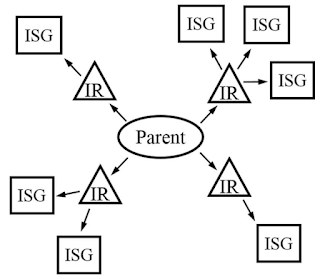Isotropy subgroup 
• Two subspaces are equivalent if the basis vectors for each can be set up in such a way that they are associated with the same representation. The reason why the two subspaces are equivalent is that it is actually the linear combination coefficients that appear in front of the basis vectors that determines how the symmetry is lowered. Even though the basis vectors of the two subspaces may be very different, the same linear combination of basis vectors still lead to the same variation of symmetry, e.g., lowering the space group from $$G$$ to its subgroup $$G'$$. This might be the reason why subgroups associated with vectors in parameter space are called isotropy subgroup -- atomic distortions in the two equivalent subspaces could be very different in 3D space (i.e., isotropic) but they both lead to the same symmetry lowering from $$G$$ to $$G'$$.